Software tools : SAS, R, Python etc Archives - Page 3 of 5 - DexLab Analytics | Big Data Hadoop SAS R Analytics Predictive Modeling & Excel VBA

## Calculating the Standard Deviation Using R & Python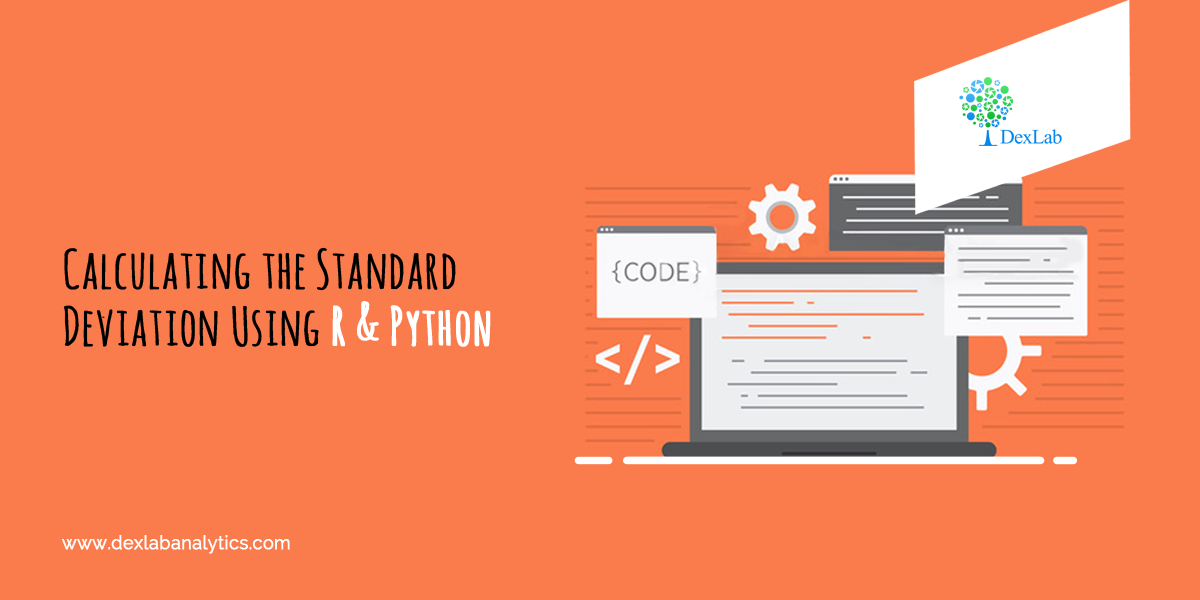When it comes to summarizing the data, standard deviation (σ) is the value which tells us about the spread of the data. More specifically, it gives information about the dispersion of each observation from the mean of the data. Now, if you are interested in understanding Mean and knowing how to calculate it, then we have shown you in CALCULATING GEOMETRIC MEAN USING R AND PYTHON And APPLICATION OF HARMONIC MEAN USING R AND PYTHON.

Thus, in essence standard deviation gives us valuable information about the robustness of the mean. The deviation is in both positive and negative direction of the mean.

Therefore, it is desirable for the standard deviation to be a low value in comparison to the mean. This would indicate a smaller spread.

Mathematically speaking, standard deviation is known as the second moment about Mean. Variance is standard deviation squared. The variance does not have any mathematical significance on its own. Think of the variance as a mere mathematical maneuver.

#### The formula for the Variance is: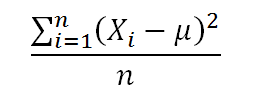Application:

An investor wants to calculate the Standard Deviation experience by his investment portfolio in last 12 months (Year 2017-2018).  The returns are:-

Month (Year 2017-18)

Returns (%)

April

12%

May

10%

June

-8%

July

4%

August

12.25%

September

18%

October

13%

November

-9%

December

-4%

January

3%

February

9%

March

11.05%#### Calculate Standard Deviation in R: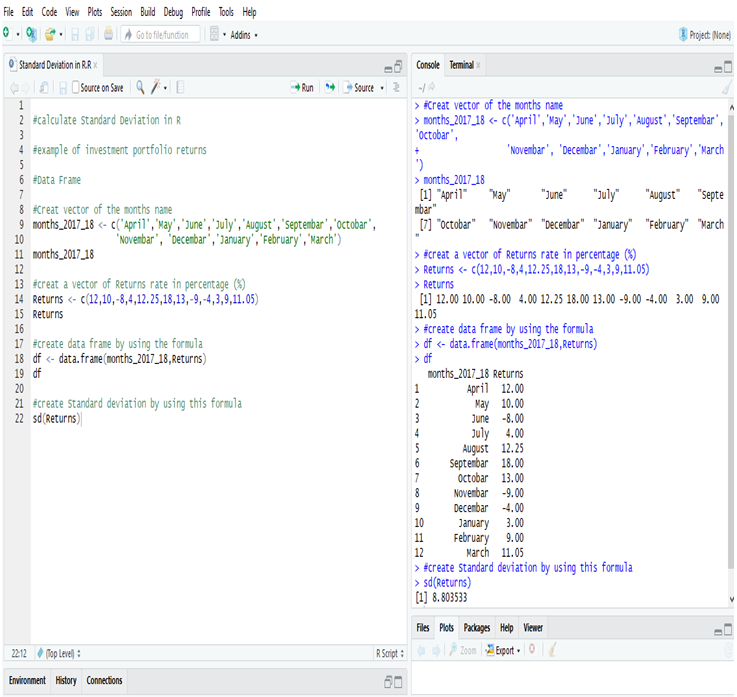Examining the Standard Deviation of the investment portfolio returns of a year in R, we get the deviation = 8.803533 or, 8.81% (Approx).

#### Calculate Standard Deviation in Python:

First, create a Data Frame in Python.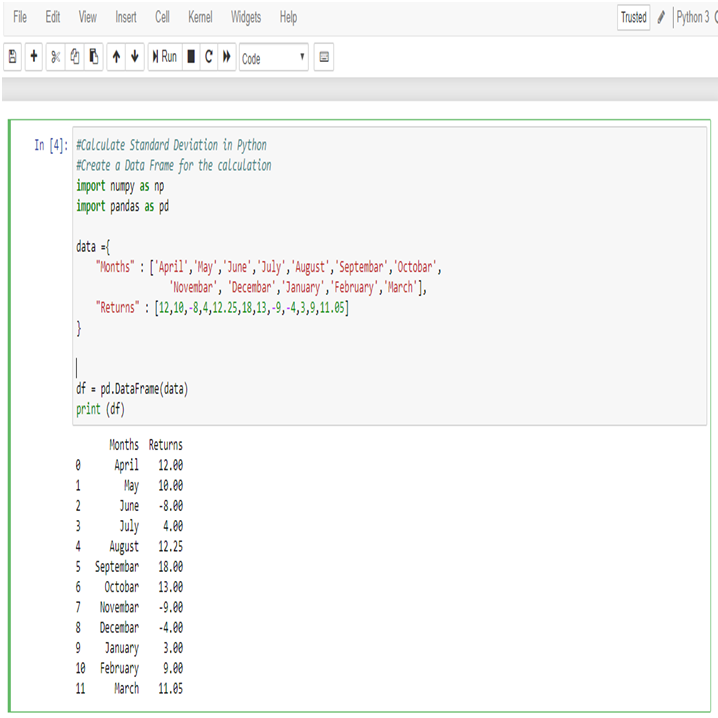Now, calculate Standard Deviation of the returns,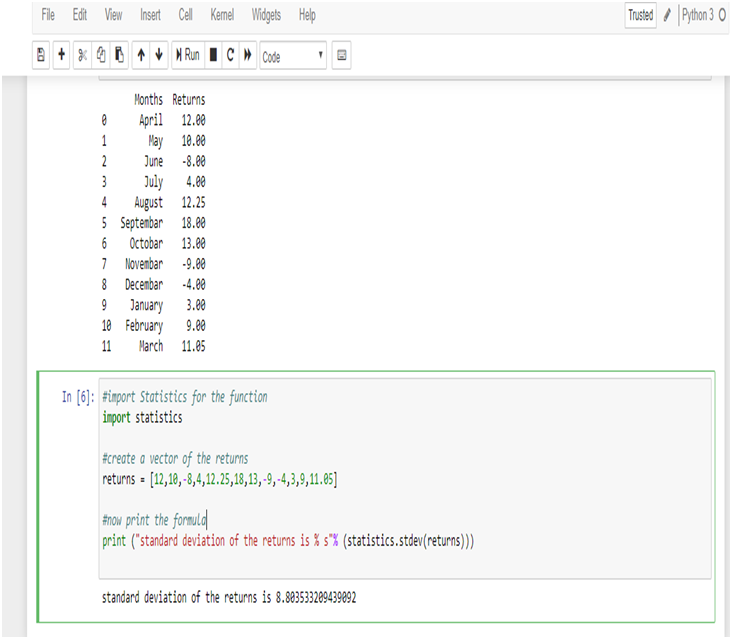Examining the Standard Deviation of the investment portfolio returns of a year in Python, we get the deviation = 8.803533209439092 or, 8.81% (Approx)

Standard Deviation is a key part of calculating margins of errors.

Standard deviation shows the variation from the mean. A low standard deviation indicates that the observations (series of number) are very close to the mean. A high standard deviation indicates that the observations (series of numbers) are spread out over a large range.

In this data the mean of the returns is 5.95%, and standard deviation is 8.81% which is close to the mean. So, the deviation of the data is low.

Thus, the investor now knows that the returns of his portfolio fluctuate by approximately 8.81% month-over-month. The information can be used to modify the portfolio to better the investor’s attitude towards risk. If the investor is risk-loving and is comfortable with investing in higher-risk, higher-return securities and can tolerate a higher standard deviation, he/she may consider adding in some small-cap stocks or high-yield bonds. Conversely, an investor who is more risk-averse may not be comfortable with this standard deviation and would want to add in safer investments such as large-cap stocks or mutual funds.

#### Endnotes

This article will surely help you to figure out the standard deviation with R and Python. However, if you want to have a general idea about Central tendency, about Mean, Median and Mode, then go through our blog on STATISTICAL APPLICATION IN R & PYTHON: CHAPTER 1 – MEASURE OF CENTRAL TENDENCY.

## Application of Median Using R And Python: Calculating Median On the Go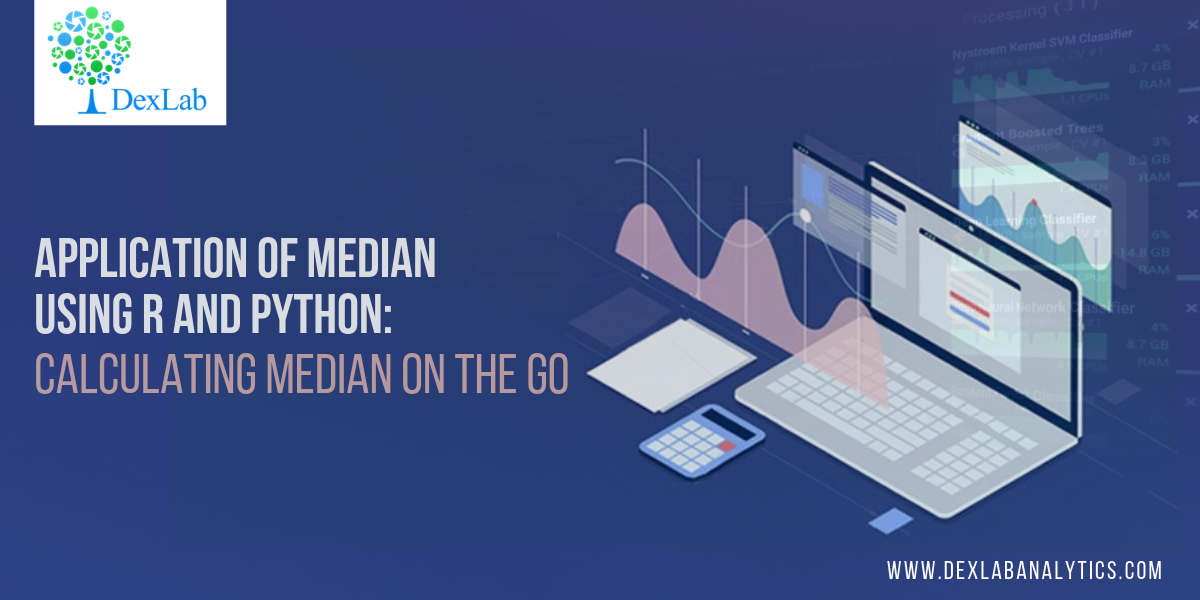This blog is in continuation of STATISTICAL APPLICATION IN R & PYTHON: CHAPTER 1 – MEASURE OF CENTRAL TENDENCY and takes you through a comprehensive way to calculate the Median in R and Python.

The term ‘Median’ is derived from the Latin word – ‘Medius’ means the center of something. In mathematics, Median is treated is that unique observation which would divide your data set into two equal halves.

If you are still unclear about Mean and/or seeking easier ways to calculate Mean using R & Python, then check

Median is special because unlike its rival, the Mean, Median is not ridiculed by the curse of extreme values. To illustrate the curse of extreme values, we bring you the following example:

In Lacs

##### 36

The mean of the above data set is: 88/7 = 12.57 lacs.

Whereas, to get the median we would have to first arrange the data into ascending order and look for the midpoint of my data i.e.,(1/2 + n/2)th observation. Where “n” is the number of observations.

The median would then be:

##### 36

Median is the 4th observation, which is 8.5 lacs.

Looking at the mean and median, it would be fair to conclude that median is the better choice to accurate summarizing the data set whenever extreme values are present. However, this may be a crude generalization which should be taken with a pinch of salt. Despite its flaws, the mean still has statistical properties used in predictive analytics which the median lacks.#### Application:

A construction company gave wages to their 10 labor (Let name A to J)  as a weekly basis, the wages are 2000, 2100, 1900, 2150, 2500, 2450, 1800, 2600, 2200, 2300. Compute the Median wages of the construction company.

Sr.NoLaborsWages (Weekly)
1A2000
2B2100
3C1900
4D2150
5E2500
6F2450
7G1800
8H2600
9I2200
10J2300

#### Calculation Median in R: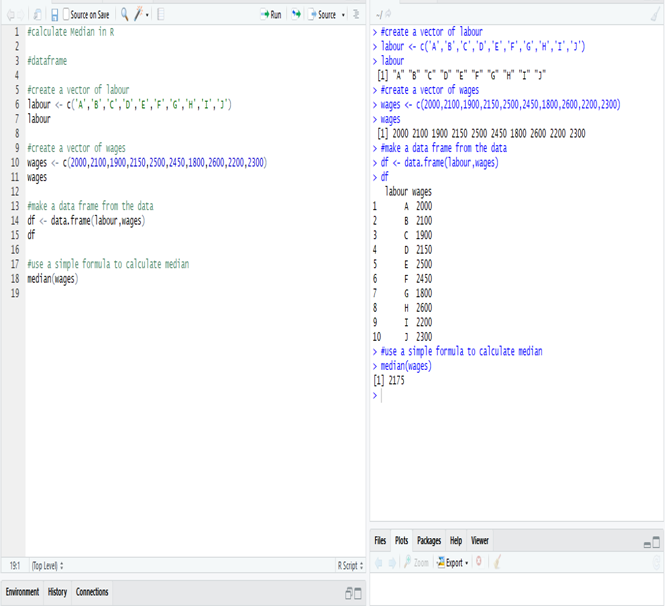The Median wage is 2175, calculate in R.

#### Calculate Median in Python:

Create a data frame of the data in Python.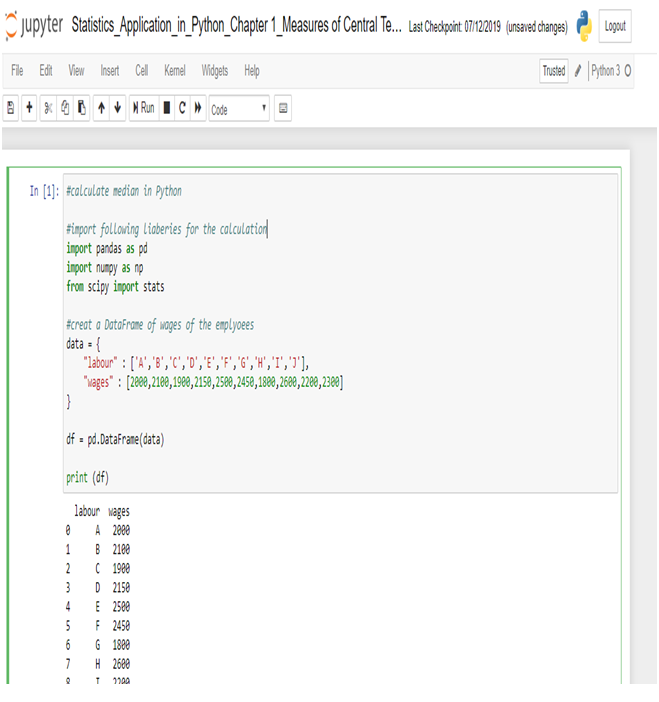Now, calculate Median in Python.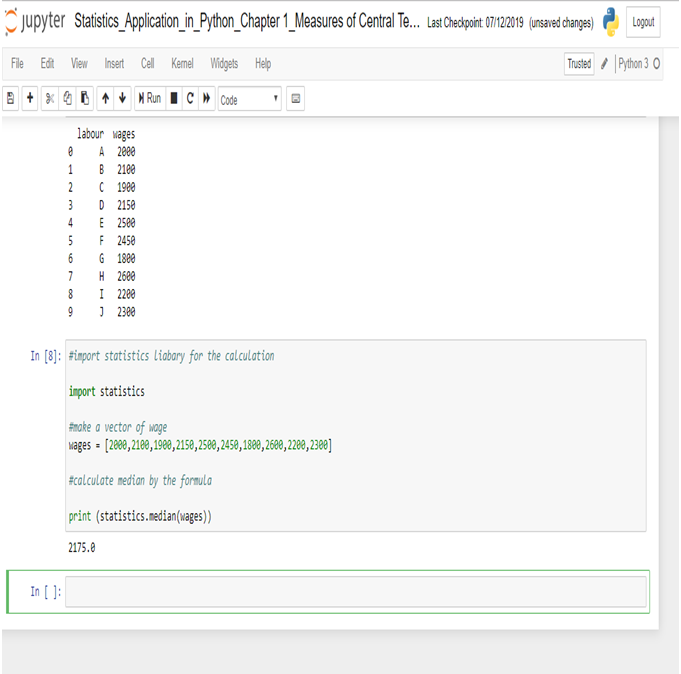The Median wage is 2175, calculated in R.

This concludes the post. If you have any queries with regards to this post, you can reach us at Dexlab Analytics. Furthermore, you can also look up for interesting and quality courses of R Programming Certification, Python Certification. Also, you can enroll with us for our combined courses of Data Science with Python Certification, Deep Learning and AI using Python, among others. So, hurry up and grab the best course!

## Application of Mode using R and Python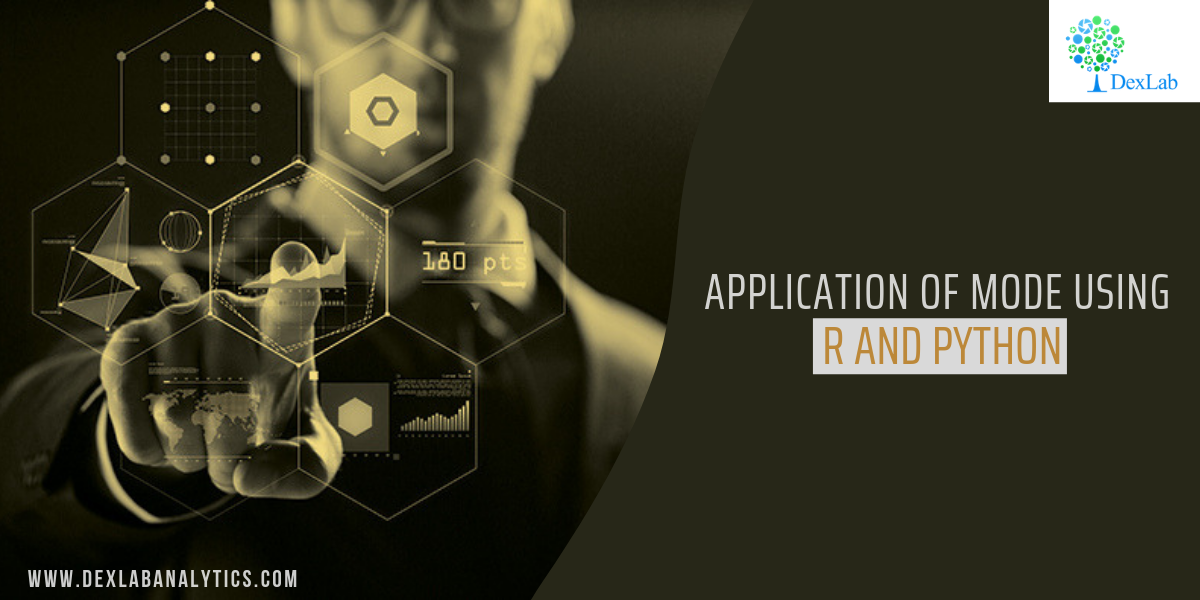Mode, for a given set of observations, is that value of the variable, where the variable occurs with the maximum or the highest frequency.

This blog is in continuation with STATISTICAL APPLICATION IN R & PYTHON: CHAPTER 1 – MEASURE OF CENTRAL TENDENCY. However, here we will elucidate the Mode and its application using Python and R.

Mode is the most typical or prevalent value, and at times, represents the true characteristics of the distribution as a measure of central tendency.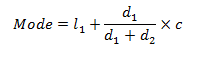#### Application:

##### 245

[Note: Here we assume total=245 when we calculate Mean from the same data]

#### Evaluate the Mode from the data.#### Calculate Mode in R: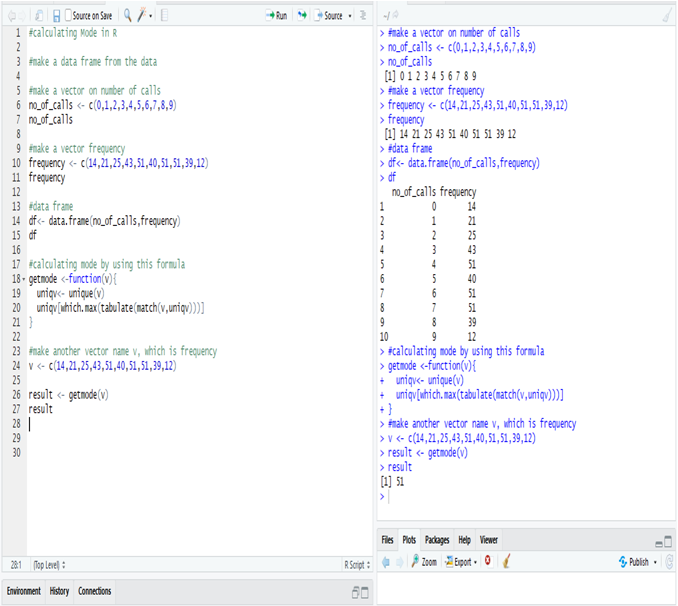Calculate mode in R from the data, i.e. the most frequent number in the data is 51.

The number 51 repeats itself in 5, 7 and 8 phone calls respectively.

#### Calculate Median in Python:

First, make a data frame for the data.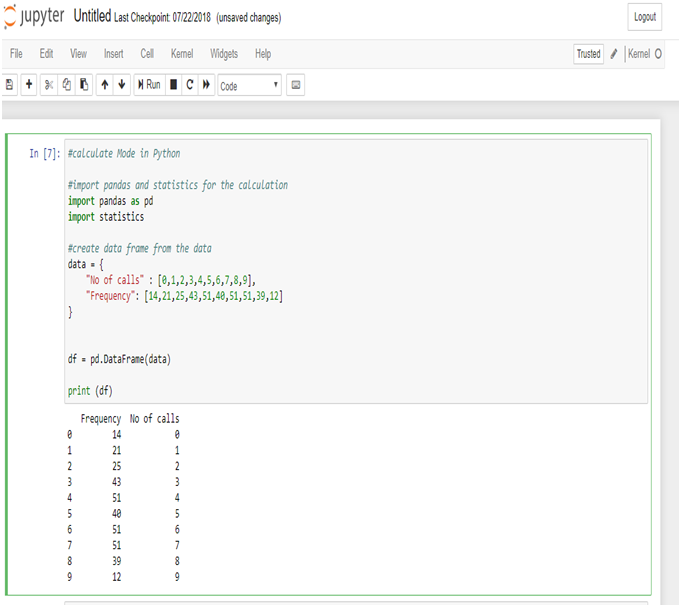Now, calculate the mode from the data frame.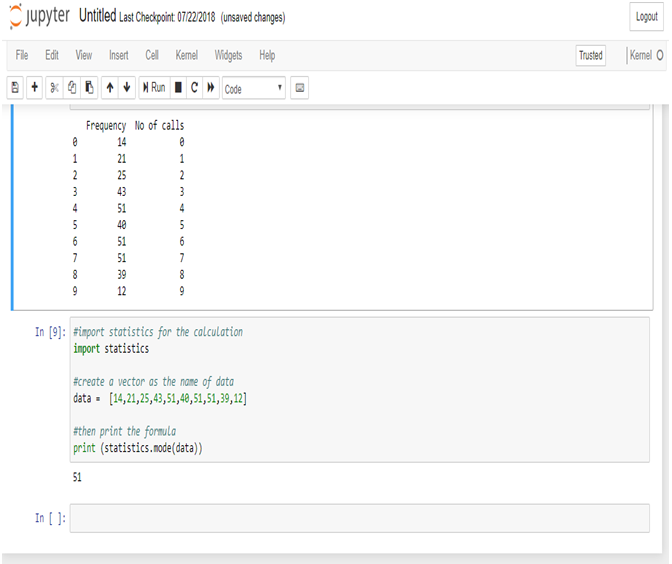Calculate mode in Python from the data, i.e. the most frequent number in the data is 51.

The number 51 repeats itself in 5, 7 and 8 phone calls respectively.

Mode is used in business, because it is most likely to occur. Meteorological forecasts are, in fact, based on mode calculations.

The modal wage of a group of the workers is the wages which the largest numbers of workers receive, and as such, this wage may be considered as the representative wage of the group.

In this particular data set we use the mode function to know the occurrence of the highest number of phone calls.

It will thus, help the Telephone Exchange to analyze their data flawlessly.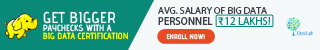Note – As you have already gone through this post, now, if you are interested to know about the Harmonic Mean, you can check our post on the

Dexlab Analytics is a formidable institute for Deep learning for computer vision with PythonHere, you would also find more information about courses in Python, Deep LearningMachine Learning, and Neural Networks which will come with proper certification at the end.

We are there in the Social Media where you can follow us both in Facebook and Instagram.

## Application of Harmonic Mean using R and Python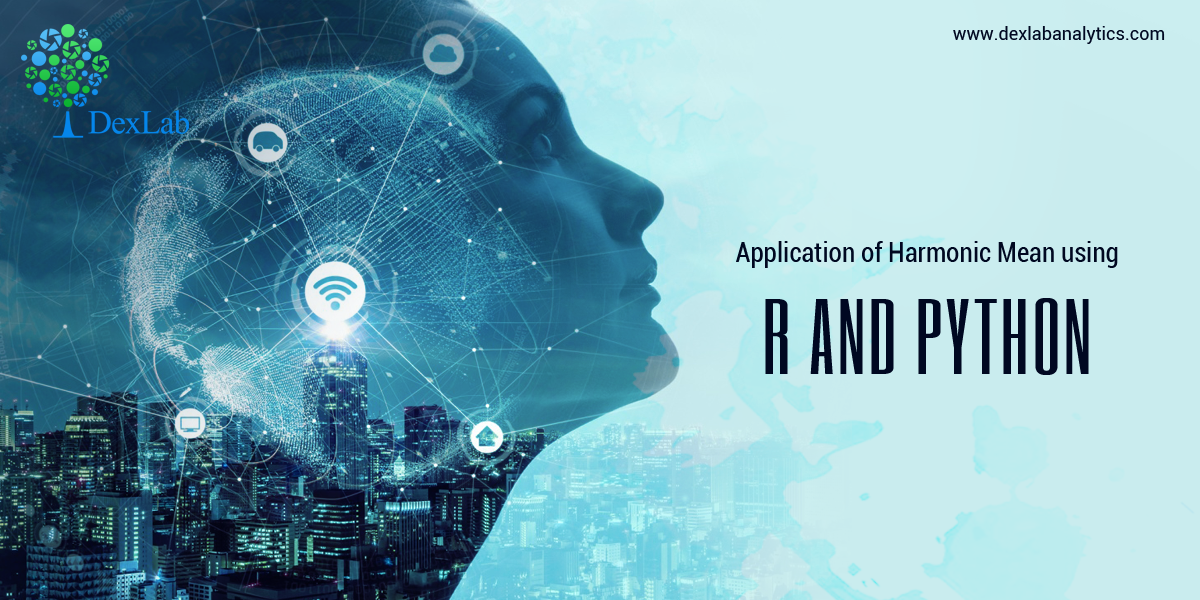Harmonic mean, for a set of observations is the number of observations divided by the sum of the reciprocals of the values and it cannot be defined if some of the values are zero.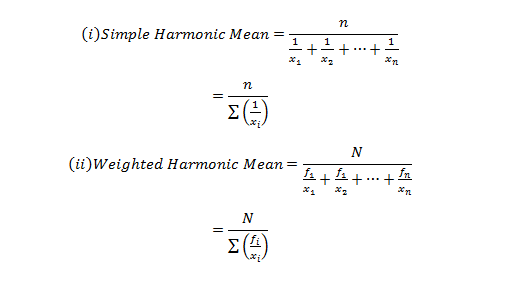This blog is in continuation with STATISTICAL APPLICATION IN R & PYTHON: CHAPTER 1 – MEASURE OF CENTRAL TENDENCY. However, here we will discover Harmonic mean and its application using Python and R.#### A milk company sold milk at the rates of 10,16.5,5,13.07,15.23,14.56,12.5,12,30,32, 15.5, 16 rupees per liter in twelve different months (January-December), If an equal amount of money is spent on milk by a family in the ten months. Calculate the average price in rupees per month.

Table for the problem:

 Month Rates (Rupees/Liter) January 10 February 16.5 March 5 April 13.07 May 15.23 June 14.56 July 12.5 August 12 September 30 October 32 November 15.5 December 16

#### Calculate Harmonic Mean in R:-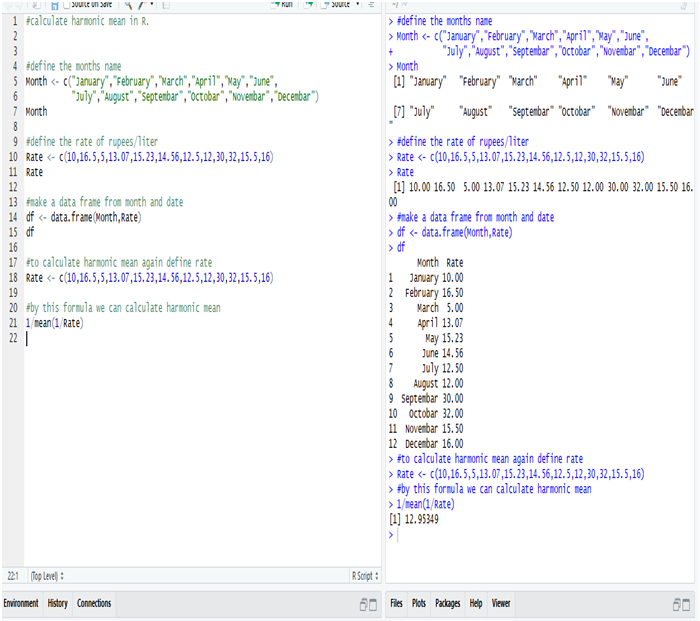So, the average rate of the milk in rupees/liter is 12.95349 = 13 Rs/liter (Approx)

We get this answer from the Harmonic Mean, calculated in R.

#### Calculate Harmonic Mean in Python:-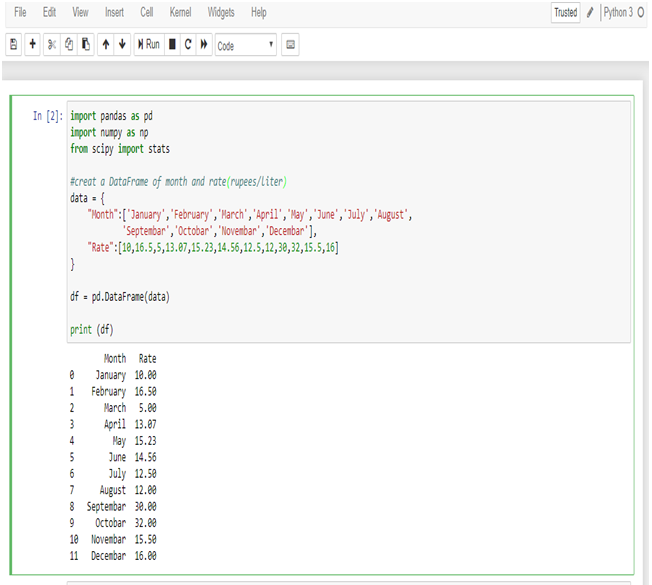First, make a data frame of the available data in Python.

Now, calculate the Harmonic mean from the following data frame.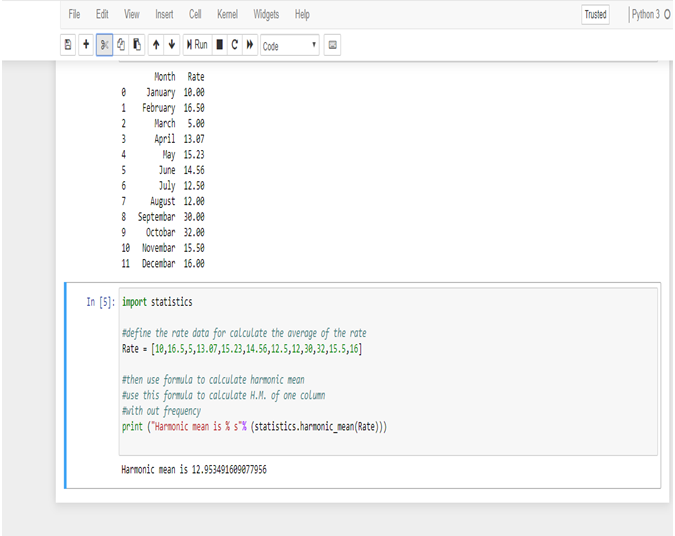So, the average rate of the milk in rupees/liter is 12.953491609077956 = 13 Rs/Liter (Approx)

We get this answer from Harmonic mean, calculated in Python.

#### Summing it Up:

In this data, we have a few large values which are putting an effect on the average value, if we calculate the average in Arithmetic mean, but in Harmonic mean, we get a perfect average from the data, and also for calculating the average rate.

Use of Harmonic mean is very limited. Harmonic mean gives the largest value to the smallest item and smallest value to the largest item.

Where there are a few extremely large or small values, Harmonic mean is preferable to Arithmetic mean as an average.

The Harmonic mean is mainly useful in averages involving time, rate & price.Note – If you want to learn the calculation of Geometric Mean, you can check our post on CALCULATING GEOMETRIC MEAN USING R AND PYTHON.

Dexlab Analytics is a peerless institute for Python Certification Training in Delhi. Therefore, for tailor-made courses in Python, Deep Learning, Machine Learning, Neural Networks, reach us ASAP!

You can even follow us on Social Media. We are available both in Facebook and Instagram.

## Python is the Leader in Data Science: Know WhyFrom being simple and effective to being updated and thereby, solving almost everything that the booming industry of Data Science of today can look up to, Python boasts of it all.

It’s not a shock that Python is finding its uses in an array of industries. It is, in fact, the language that the Data Scientists rely on. Thus, our tailored courses of Python Certification Training in Delhi would be helpful for all in this digital age.

Let’s see some more of the advantages for which Python stands distinguished among the other programming languages:

#### Handling Data without a Hassle

The field of Data Science is entrusted with the handling of incredibly large amounts of data which is found to be intricate to compute. However, with Python, it is now simpler than ever. Any of the other high-level programming languages would make it rather difficult and messy compared to the peerless Python, if we talk about analytical and quantitative computing.

#### Open Source Programming Language

Python is an open-source programming language. Wonder why this programming language is the most preferred still?

It truly opens a whole lot of opportunities that the language can build upon, being open-source in nature. Furthermore, there is not a single restriction regarding Python. Thus, you can be as creative as you wish on this programming language.

#### It is Powerful and Easy to Use

Python is an easy language right from the start for which it has become so popular. Any of the beginners with just the rudimentary knowledge can start fine with Python. Besides, once you are on with this programming language, you can start progressing with it day by day at your own pace.

The implementation of the code has a slower approach in the languages: Java, C and C#, but if you try Python, you would discover that it is fast to debug and effective to perform. The prompt results in coding would aid with an added boost in your work.

#### In the Library of Python

Python is an all-absorbing language that even supports the cutting edge technologies of Machine Learning and Artificial Intelligence. And on top of it, Python also offers its users a colossal database of libraries. Therefore, you can simply check in the libraries, import them and then implement all of them in your day to day coding.

#### It is Highly Scalable

In the parameter of scalability, Python superbly stands out. The programming languages: R and Java certainly falls short in this factor. Thus, with the ease of scalability and quicker turnaround times, data scientists and nearly all of the organisations exploring Data Science, are choosing Python over any other existing languages.#### It is Peerless in Visualisation and Graphics

As the smooth rendering of quality graphics and visualisation is the demand of the age, Python fits in quite comfortably here. With an exhaustive range of options for visualisation, which are simple and efficient, the world of Data Science is rooting for Python.

With all the benefits that you can reap, Python for data analysis is a must, if you want to be absorbed in the industry of Data Science.

.

## Calculating Geometric Mean Using R and Python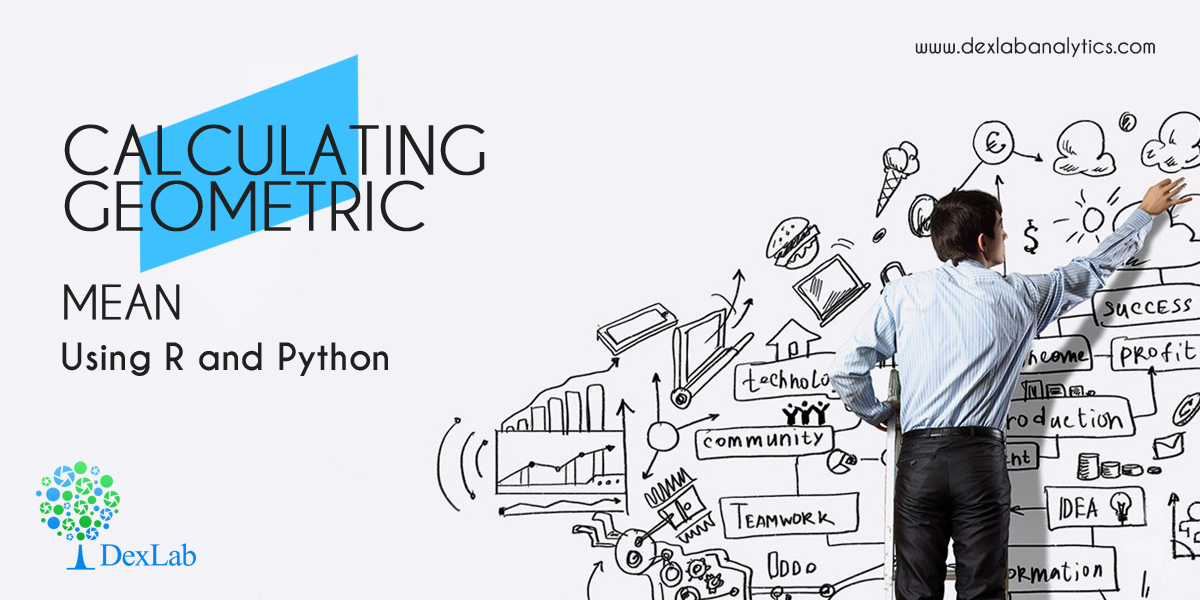In this blog, we are going to discuss the Geometric Mean and its application using Python and R.

Geometric Mean of group of ‘n’ observations is the nth root of their product. It is defined only when all observations have the same sign and none of them is zero.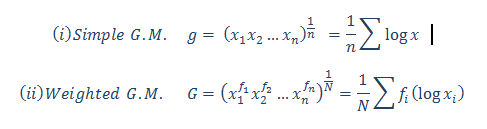#### Calculate the Geometric Mean of the salary increment of 12 employees. From the following table, calculate the average salary increment of the year (2019-2020):-

 Name Salary Increment inPercentage (%) Ritesh 10.09% Heena 15.45% Kritika 9% Anuradha 13.06% Gaurav 20% Prakash 14% Aarti 16% Meena 6.25% Utkarsh 12.85% Chirag 10% Neha 18% Smrita 21.36%

#### Calculate the Geometric Mean in R: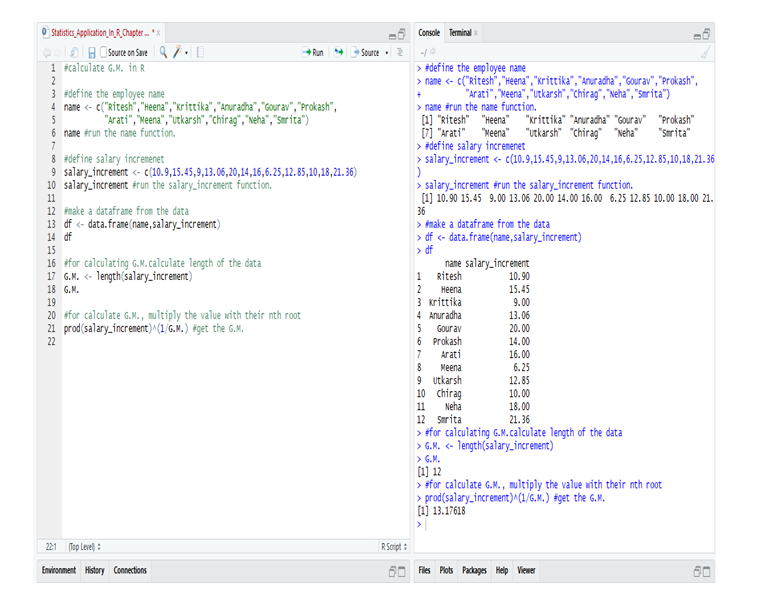So, from the data of the employee’s in R we calculate the G.M. and get that the average salary increment in the year (2019-2020) = 13.17618 or 13.18% (approx).

#### Calculate the Geometric Mean in Python: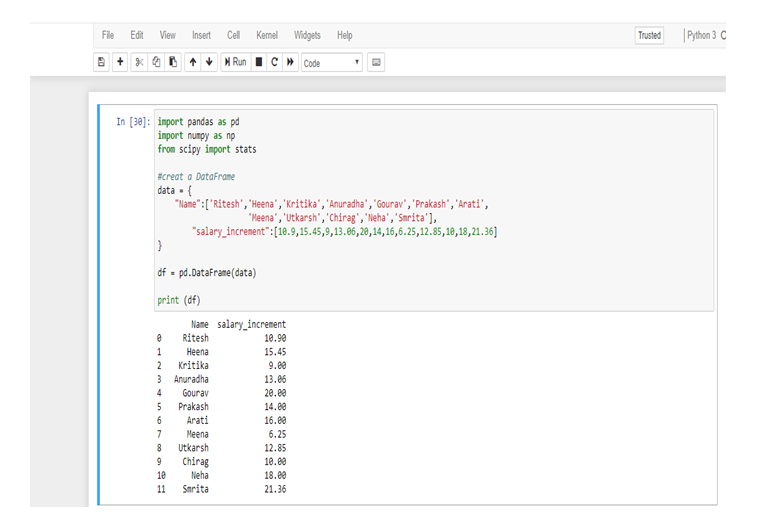First, make a data frame in Python from the following table.

Now, calculate the Geometric Mean from the data-frame.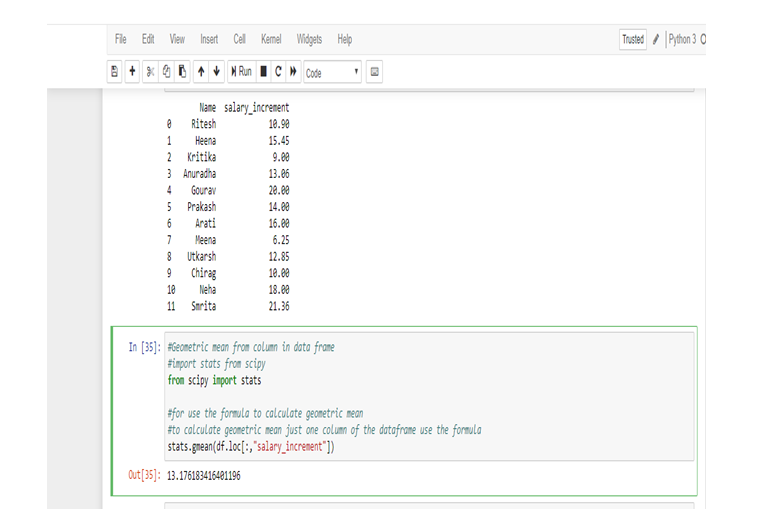So, from the data of the employee’s in Python we calculate the G.M. and get that the average salary increment in the year (2019-2020) = 13.176183416401196 or 13.18% (approx).

We use Geometric Mean for calculating ratios, rates and percentages. And it is not affected by the extreme value or outlier. In this particular problem, we use Geometric Mean because an average of the salary increment of the employee’s not affected by the extreme highest or extreme lowest value, that’s why the salary increment rates of Meena and Smrita do not have any effect on the total average rate.

Geometric Mean gives small value than Arithmetic Mean.Note: This is a continuation of the blog: Statistical Application in R & Python: Chapter 1 – Measure of Central Tendency. It would be better to go through the first installment and then read this one. More blogs are to be followed, so stay tuned.

DexLab Analytics is a premier Python training institute in Delhi. Our industry-relevant courses are carefully crafted by experts. Follow us on Facebook and Instagram.

## Statistical Application In R & Python: Chapter 1 – Measure Of Central Tendency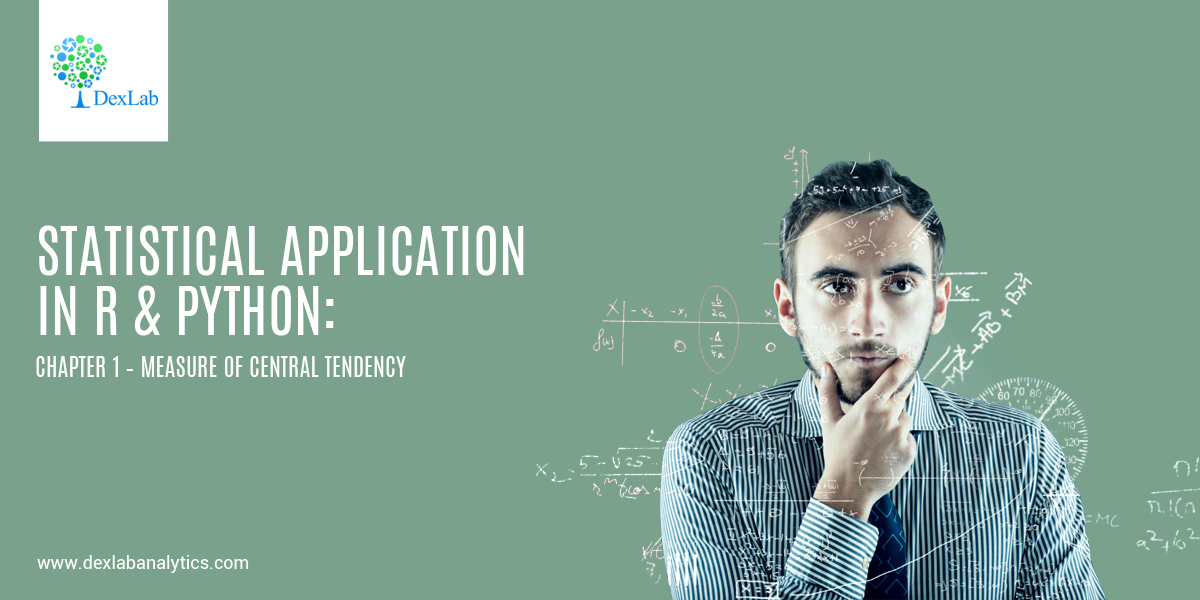Statistical analysis helps explore data relationship and develop high-end models to frame better decisions. It’s an intricate process of collecting and evaluating data to define the nature of data that has to be analyzed.

Below, we dig into the basics of statistical application in R and Python using the measure of central tendency.

• #### Introduction:-

As body methods for the study of numerical data, if some rows or columns are too long, in such cases, it becomes necessary to summarize data in an easily manageable form. The purpose is to serve by classifying the data in the form of frequency distribution and various graphs. When data relate to a variable, the process of summarization can be taken a step further by using certain descriptive measures. The dim is to focus on certain features that are central frequency and description.• #### Central Tendency :

In a set of data, they have a tendency, notwithstanding their variability, to cluster-around a central value and the tendency of the quantitative statistical observations is called central tendency.

The three measures of the central tendency are commonly used is:-

• Mean
• Median
• Mode

The description of these 3 estimators start below:-

• #### Mean:-

Mean is the average of central tendency and is the most commonly used measures.

The concept of mean is divided into three parts:-

• Arithmetic mean.
• Geometric mean.
• Harmonic mean.

Mainly the mean refers to an arithmetic mean.

• #### Arithmetic Mean (A.M.):-

The arithmetic mean of a set of observations is defined to be their sum, divided by the number of observations.

For n numbers of observation (x1,x2,… ,xn )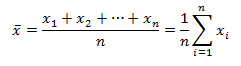• #### Weighted A.M.

For frequency distribution where  have  frequencies. (i=1,2,3…)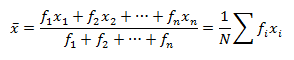• #### Application of A.M.:-

Let’s, calculate the mean of Age, Height & Weight from the given data.

 Name Sex Age Height Weight Ritesh M 24 6.9 112.5 Heena F 23 5.65 84 Kritika F 23 6.53 98 Anuradha F 24 6.28 102.5 Gaurav M 24 6.35 102.5 Prakash M 22 5.73 83 Aarti F 22 5.98 84.5 Meena F 25 6.25 112.5 Utkarsh M 23 6.25 84 Chirag M 22 5.9 99.5 Neha F 21 5.13 50.5 Smrita F 24 6.43 90

#### Calculating Mean in Python: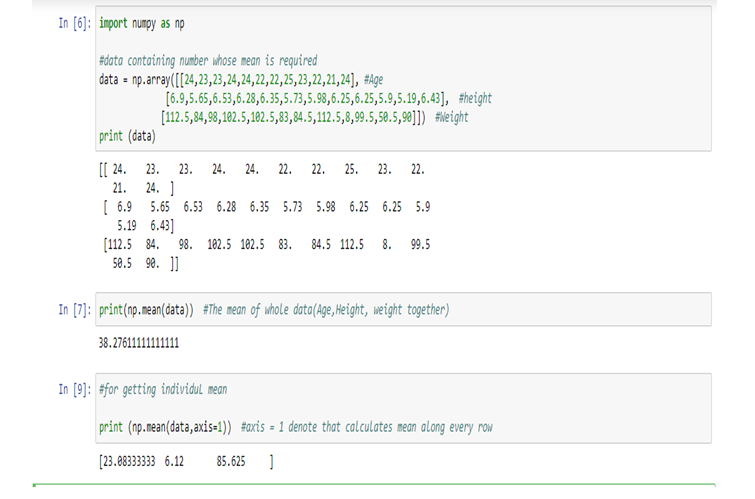Therefore,

Age (Mean) = 23.08333333, Height (Mean) = 6.12, weight(Mean) = 85.625

#### Calculating Mean in R: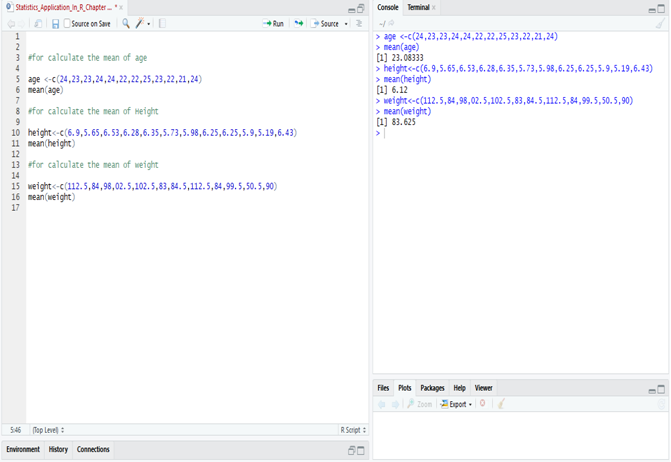• #### Application of Weighted A.M.:-

The weighted mean is denoted that the mean with frequency.

#### Calculate the average price per ton of coal purchased by the industry for the half-year.

 Month Price Per Ton Tons Purchased January Rs. 52.49 26 February Rs. 62.23 34 March Rs. 87.26 40 April Rs. 45.25 54 May Rs. 78.56 13 June Rs. 69.25 45

#### Data to solve:

 Month Price (Rs)Per Ton(x) TonsPurchased(f) fx=y(Main Data) January 52.49 26 1364.74 February 62.23 34 2115.82 March 87.26 40 3490.4 April 45.25 54 2443.5 May 78.56 13 1021.28 June 69.25 45 3116.25 Total 395.04 N=212 13551.99

The price is denoted as x (52.49, 62.23, 87.26, 45.25, 78.56, 69.25 [in Rs.])=395.04

The amount of purchased (frequency) is denoted by f (26, 34, 40, 54, 13, 45) = 212 (N)

Then multiply the x and f and we get the total amount which is denoted by y, fx(y) = 13551.99

#### Calculate Weighted Mean in R: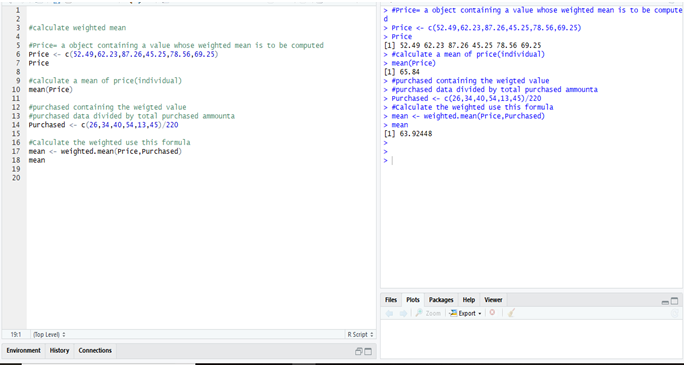#### Calculate Weighted Mean in Python: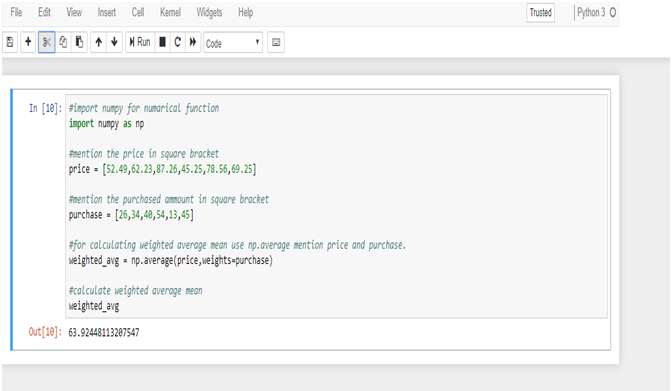To calculate the weighted mean from R & Python we get the same result = 63.9244811.

Want to know more about the nature of data? Keen to perform high-end statistical analysis using Python and R? Follow DexLab Analytics, an excellent Python training center in Gurgaon, India. Our team of consultants will help you learn the basics of R and Python in the easiest manner possible.

## Top 4 Python Industrial Use-Cases: Explained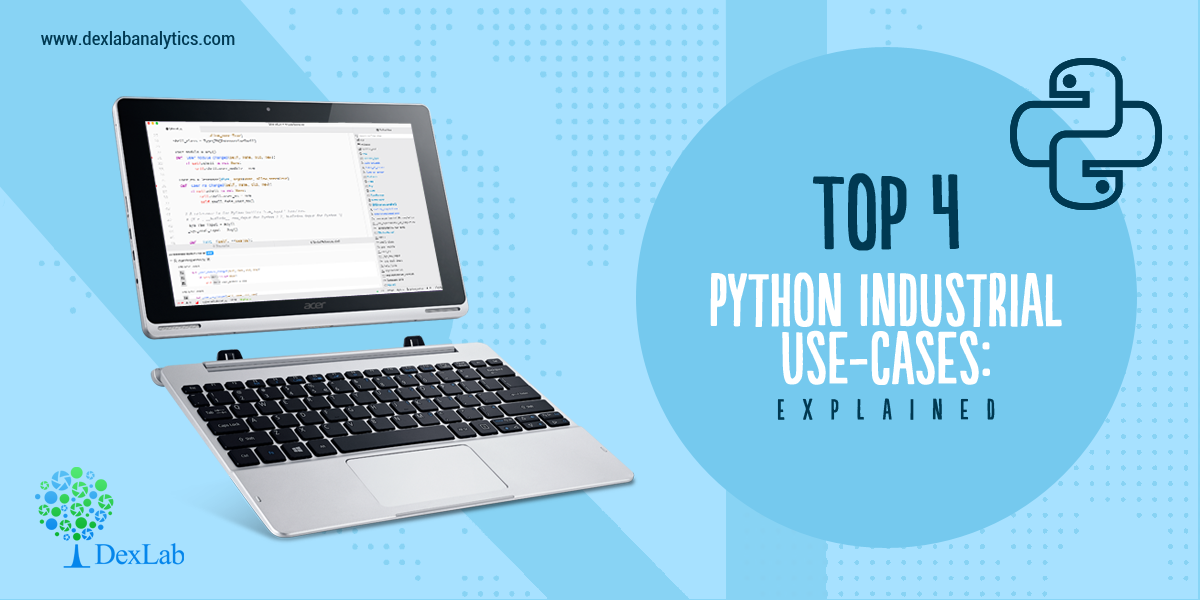Python is one of the fastest-growing and most popular coding languages in the world; a large number of developers use it on daily basis and why not, it works brilliantly for a plethora of developer job roles and data science positions – starting from scripting solution for sysadmins to supporting machine learning algorithms to fueling web development, Python can work wonders across myriad platforms!

Below, we’ve rounded up 4 amazing Python industrial use-cases; scroll ahead:

#### Widely used in generating business insights; courtesy machine learning.

Case Study:

Smaller firms driven by machine learning gave stiff competition to a US multinational finance and insurance corporation. In return, the insurer formed teams and devised a new set of services and applications based on ML algorithms to enjoy a competitive edge. However, the challenge was that with so many data science tools, numerous versions of Python came into the picture and gave rise to compatibility issues. As a result, the company finalized only one version of Python, which was then used in line with machine learning algorithms and tools to derive specific results.#### Data mining helps determine cross-sell opportunities.

Case Study:

Another US MNC dealing in financial services showed interest in mining complex customer behavioral data. Using Python, the company launched a series of ML and data science initiatives to dig into its structured data that it has been gathering for years and correlated it with an army of unstructured data, gathered from social media and web to enhance cross-selling and retrieve resources.

#### Python helps in meeting system deadlines and ensured utmost confidentiality.

Case Study:

Recently, the International Space Station struck a deal with an American MNC dealing in military, defense and aerospace technology; the latter has been asked to provide a series of systems to the ISS. The critical safety systems were mostly written in languages, like Ada; they didn’t fare well in terms of scripting tasks, data science analysis or GUI creation. That’s why Python was chosen; it offered bigger contract value and minimum exposure.

#### Enjoy flexible data manipulation and transformation – all with Python!

Case Study:

A top-notch US department store chain equipped with an in-store banking division gathered data and stored it in a warehouse. The main aim of the company was to share the information with multiple platforms to fulfill its supply chain, analytics, retail banking and reporting needs. Though the company chose Python for on-point data manipulation, each division came up with their own versions of Python, resulting in a new array of issues. In the end, the company decided to keep a standard Python; this initiative not only resulted in amplifying engineering speed but also reduced support costs.

As end notes, Python is the next go-to language and is growing each day. If you have dreams of becoming an aspiring programmer, you need to book the best Python Certification Training in Delhi. DexLab Analytics is a premier Python training institute in Delhi; besides Python, it offers in-demand skill development courses for interested candidates.

The blog has been sourced from www.techrepublic.com/article/python-5-use-cases-for-programmers

## General Python Guide 2019: Learning Data Analytics with Python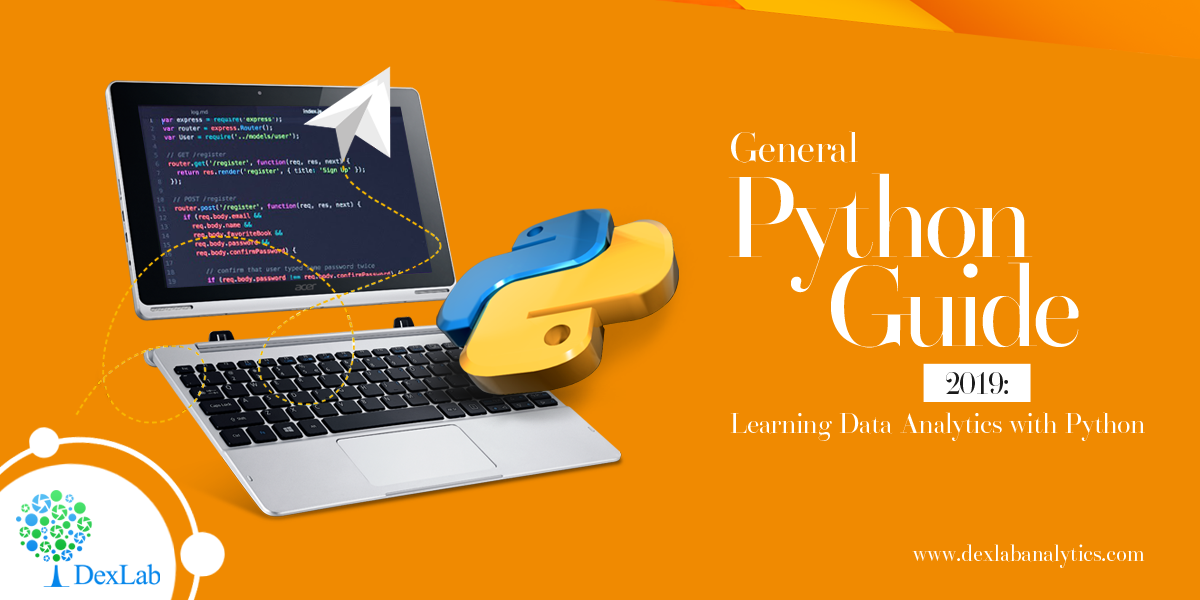Python and data analytics are possibly three of the most commonly heard words these days. In today’s burgeoning tech scene, being skillful in these two subjects can prove very profitable. Over the years, we have seen the importance of Python education in the field of data science skyrocketing.

So here we present a general guide to help start off your Python learning:

• #### Popularity

With over 40% data scientists preferring Python, it is clearly one of the most widely used tools in data analysis. It has risen in popularity above SAS and SQL, only lagging behind R.

• #### General Purpose Language

There might be many other great tools in the market for analyzing data, like SAS and R, but Python is the only trustworthy general-purpose language valid across a number of application domains.#### Step 1: Setup Python Environment

Setting up Python environment is uncomplicated, but a primary step. Downloading the free Anaconda Python package is recommended. Besides core Python language, it includes all the essential libraries, such as Pandas, SciPy, NumPy and IPython, and graphical installer also. Post installation, a package containing several programs is launched, most important one being iPython also known as Jupyter notebook. After launching the notebook, the terminal opens and a notebook is started in the browser. This browser works as the coding platform and there’s no need for internet connection even.

#### Step 2: Knowing Python Fundamentals

Getting familiar with the basics of Python can happen online. Active participation in free online courses, where video tutorials, practice exercises are plentiful, can help you grasp the fundamentals quickly. However, if you are seeking expert guidance, you must explore our Python data science courses.

#### Step 3: Know Key Python Packages used for Data Analysis

Since it is a general purpose language, Python’s utility stretches beyond data science. But there are plentiful Python libraries useful in data functionalities.

Numpy – essential for scientific computing

Matplotib – handy for visualization and plotting

Pandas – used in data operations

Skikit-learn – library meant to help with data mining and machine learning activities

StatsModels – applied for statistical analysis and modeling

Scipy-SciPy – the Numpy extension of Python; it is a set of math functions and algorithms

Theano – package defining multi-dimensional arrays.

#### Step 4: Load Sample Data for Practice

Working with sample datasets is a great way of getting familiar with a programming language. Through this kind of practice, candidates can try out different methods, apply novel techniques and also pinpoint areas of strength and in need of improvement.

Python library StatModels contains preloaded datasets for practice. Users can also download dataset from CSV files or other sources on web.

#### Step 5: Data Operations

Data administration is a key skill that helps extract information from raw data. Majority of times, we get access to crude data that cannot be analyzed straightaway; it needs to be manipulated before analyzing. Python has several tools for formatting, manipulating and cleaning data before it is examined.

#### Step 6: Efficient Data Visualization

Visuals are very valuable for investigative data analysis and also explaining results lucidly. The common Python library used for visualization is Matplotlib.

#### Step 7: Data Analytics

Formatting data and designing graphs and plots are important in data analysis. But the foundation of analytics is in statistical modeling, data mining and machine learning algorithms. Having libraries like StatsModels and Scikit-learn, Python provides all necessary tools essential for performing core analyzing functions.

#### Concluding

As mentioned before, the key to learning data analytics with Python is practicing with imported data sets. So without delay, start experimenting with old operations and new techniques on data sets.

For more useful blogs on data science, follow DexLab Analytics – we help you stay updated with all the latest happenings in the data world! Also, check our excellent Python courses in Delhi NCR.